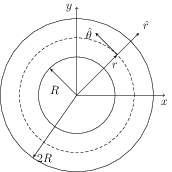# Magnetic Field Inside a Long Straight Solenoid

## Problems from IIT JEE

Problem (IIT JEE 2013): A steady current $I$ flows along an infinitely long hollow cylindrical conductor of radius $R$. The cylinder is placed coaxially inside an infinite solenoid of radius $2R$. The solenoid has $n$ turns per unit length and carries a steady current $I$. Consider a point P at a distance $r$ from the common axis. The correct statement(s) is (are)

1. In the region $0 < r < R$, the magnetic field is non-zero.
2. In the region $R < r < 2R$, the magnetic field is along the common axis.
3. In the region $R < r < 2R$, the magnetic field is tangential to the circle of radius $r$ centered on the axis.
4. In the region $r > 2R$, the magnetic field is non-zero.

Solution:Let $\vec{B}_{\textrm{cyl}}$ be the magnetic field due to the hollow cylindrical conductor of radius $R$, $\vec{B}_{\textrm{sol}}$ be the magnetic field due to the solenoid of radius $2R$, and $\vec{B}_{\textrm{net}}$ be the resultant of these two. The direction of $\vec{B}_\text{cyl}$ is tangential (along $\hat{\theta}$) and its magnitude remains same at all points lying on a circle of radius $r$ (see figure). The Ampere's circuital law, $\oint\vec{B}\cdot\mathrm{d}\vec{l}=\mu_0I_{\textrm{enc}}$, gives field due to the cylindrical conductor as, \begin{alignat}{2} \label{xvb:eqn:1} \vec{B}_{\textrm{cyl}}= \begin{cases} 0, & \text{if $r < R$;}\\ \frac{\mu_0 I}{2\pi r}\,\hat{\theta}, & {\text{otherwise.}} \end{cases} \end{alignat} The field due to the solenoid is given by, \begin{alignat}{2} \label{xvb:eqn:2} \vec{B}_{\textrm{sol}}= \begin{cases} \mu_0 n I\, \hat{z}, & \text{ if $r < 2R$;} \\ 0, & \text{otherwise.} \end{cases} \end{alignat} These equations gives net field as, \begin{alignat}{2} \vec{B}_{\textrm{net}}= \begin{cases} \mu_0 n I\, \hat{z}, & \text{ if $r < R$;} \\ \mu_0 n I\, \hat{z}+\frac{\mu_0 I}{2\pi r}\,\hat{\theta}, & \text{ if $R < r < 2R$;} \\ \frac{\mu_0 I}{2\pi r}\,\hat{\theta}, & \text{otherwise.} \end{cases} \nonumber \end{alignat}